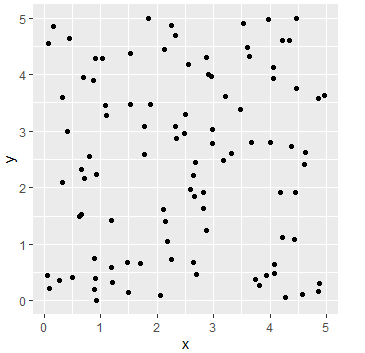3 views

I'm trying to resize a plot to fit into my document, but I'm having difficulties getting the plotted diagram do be a square.

Example:

pdf(file = "./out.pdf", width = 5, height = 5)

p <- ggplot(mydata, aes(x = col1, y = col2))

print(p)

aux <- dev.off()

Although the limits for x and y are the same, the plot in the result isn't square. I guess that R makes the enclosing panel 5x5" but doesn't care about the actual diagram size.

How can I unsquash my diagrams?

by

To preserve the aspect ratio of your plot In ggplot2 you can add a coord_fixed() layer to the plot. This preserves the aspect ratio of the plot itself, regardless of the shape of the actual bounding box.

library(ggplot2)

df <- data.frame(

x = runif(100, 0, 5),

y = runif(100, 0, 5))

ggplot(df, aes(x=x, y=y)) + geom_point() + coord_fixed()